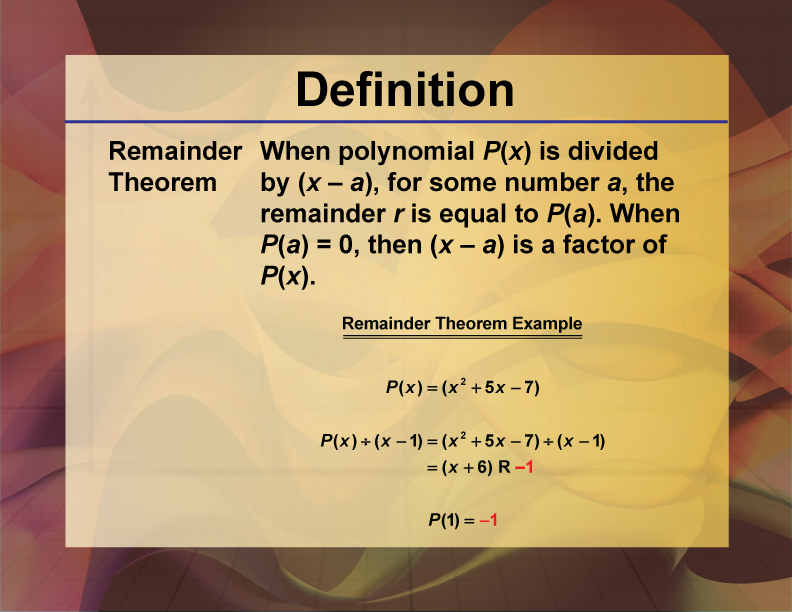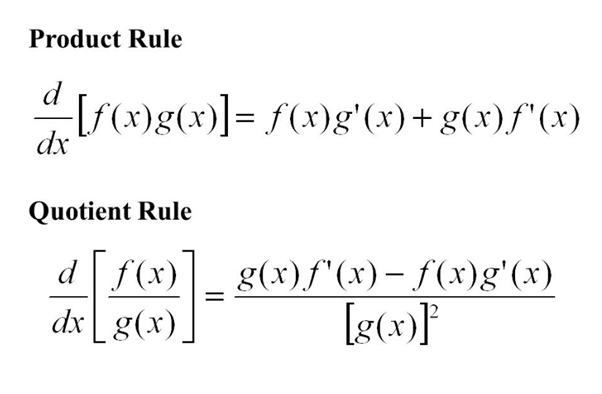## Remainder Theorem, Definition, Formula and Examples

The remaining theorem is a formula for calculating the remainder when dividing a polynomial by a linear polynomial. The amount of items left over after dividing a specific number of things into groups with an equal number of things in each group is known as…## What are quotient and product rules? What are the derivatives and their uses?

Quotient rule is a formulaic application one uses in differentiation problems. So, in this case, one function divides another. There is a particular way in which you must remember the quotient rule. So, begin with the bottom function and end by squaring the same. You…